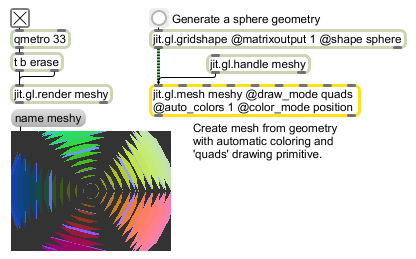jit.gl.mesh

Generates GL geometry from existing data

Description

The jit.gl.mesh object creates a geometric surface from a jit.matrix connected to the left-most inlet containing spatial coordinates. Additional geometry can be specified by attaching other jit.matrix objects to the other inlets, which correspond to (from left to right): vertex, texcoord, normal, color, specular, edgeflag, tangent, bitangent, and index data.
The jit.gl.mesh object requires one argument: the name of a drawing context. A named drawing context is a named instance of a jit.window, jit.pwindow, or jit.matrix object that has an instance of the jit.gl.render object associated with it. This value may also be set via the OB3D drawto attribute.

Messages

 color_matrix Specifies a matrix of color values. This must be the same size as the matrix specified by the vertex_matrix message, and can be a 3 or 4 plane matrix. bind Binds the internal mesh buffers for drawing. bitangent_matrix The word bitangent_matrix, followed by a symbol, specifies a matrix of bitangent values. This must be the same size as the matrix specified by the vertex_matrix message. It must be a 3 plane matrix. edgeflag_matrix The word edgeflag_matrix, followed by a symbol, specifies a matrix of edge flag values. This must be the same size as the matrix specified by the vertex_matrix message. It must be a 1 plane matrix. get_cache The word index_matrix, followed by a symbol, matrix of indices. Although it can be any size, it must be a 1 plane integer matrix. index_matrix The word index_matrix, followed by a symbol, specifies a matrix of indices. It can be any size, but it must have 1 plane and be an integer matrix. normal_matrix The word normal_matrix, followed by a symbol, specifies a matrix of normal values. This must be the same size as the matrix specified by the vertex_matrix message. It must be a 3 plane matrix. reset The word reset resets all parameters to their default states. texcoord_matrix The word texcoord_matrix, followed by a symbol, specifies a matrix of texture coordinate values. This must be the same size as the matrix specified by the vertex_matrix message. It can be a 2, 3, or 4 plane matrix. specular_matrix The word specular_matrix, followed by a symbol, specifies a matrix of specular values tangent_matrix The word tangent_matrix, followed by a symbol, specifies a matrix of tangent values. This must be the same size as the matrix specified by the vertex_matrix message. It must be a 3 plane matrix. vertex_attr_matrix The word vertex_attr_matrix, followed by a symbol, specifies a matrix of arbitrary per-vertex data vertex_matrix The word ertex_matrix, followed by a symbol, specifies a matrix of vertex values. It can be 3 or 4 planes. unbind Unbinds the internal mesh buffers after drawing.

Attributes

Name Type g/s Description
auto_bitangents int Enables or disables the calculation of bitangent (aka binormal) directions (only available if texcoords and normals exist) (default = 0).
auto_colors int Enables or disables the calculation of vertex colors (default = 0).
auto_normals int Enables or disables the calculation of normal directions used for calculating lighting (default = 1).
auto_tangents int Enables or disables the calculation of tangent directions (only available if texcoords and normals exist) (default = 0).
cache_mode symbol The mode to use for the geometry cache. (default = var) Supported modes are:
none
var (vertex arrays)
vbo (vertex buffer objects)
color_mode symbol The mode to use for the calculating vertex colors. (default = none) Supported modes are:
index
position
vertex
normal
texcoord
edgeflag
tangent
bitangent
none
draw_mode symbol The mode to use for rendering the geometry. (default = tri_grid) Supported modes are:
points
point_sprite
lines
line_strip
line_loop
triangles
tri_strip
tri_fan
polygon
tri_grid

ExamplesName Description
jit.gl.graph Open GL floating-point data visualization
jit.gl.gridshape Generate simple geometric shapes as a connected grid
jit.gl.handle Use mouse movement to control position/rotation
jit.gl.isosurf Generates a GL based surface extraction
jit.gl.model Read and draw Wavefront .obj models
jit.gl.nurbs Generate NURBS surface
jit.gl.plato Generate platonic solids
jit.gl.render Render Open GL